C Program to Add n Numbers

In this tutorial you will learn about the C Program to Add n Numbers and its application with practical example.

C Program to Add n Numbers

In this tutorial, we will learn to create a C program that will Add n Numbers using C programming.

Prerequisites

Before starting with this tutorial we assume that you are best aware of the following C programming topics:

• Operators in C Programming.
• Basic Input and Output function in C Programming.
• Basic C programming.
• For loop in C programming.
• Arithmetic operations in C Programming.

In c programming, it is possible to take numerical input from the user and Add n Numbers with the help of a very small amount of code. The C language has many types of header libraries which has supported function in them with the help of these files the programming is easy. In C language, we can do many arithmetic operations.

With the help of this program, we can Add n Numbers.

Here,

We will first take the input

The second will make a series and add the numbers.

Output:-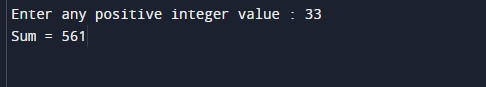In the above program, we have first initialized the required variable.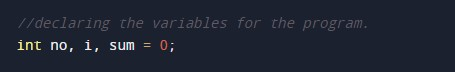• no = it will hold the integer value.
• i = it will hold the integer value.
• sum = it will hold the integer value.

Input number from the user.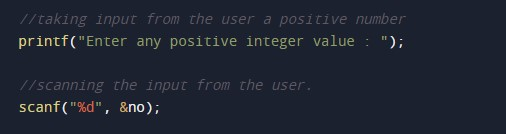Program Logic Code.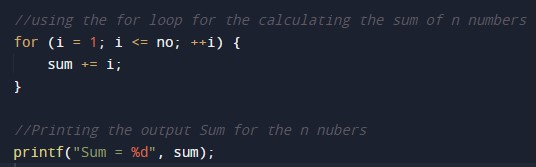Printing output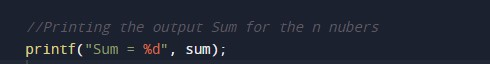In this tutorial we have learn about the C Program to Add n Numbers and its application with practical example. I hope you will like this tutorial.